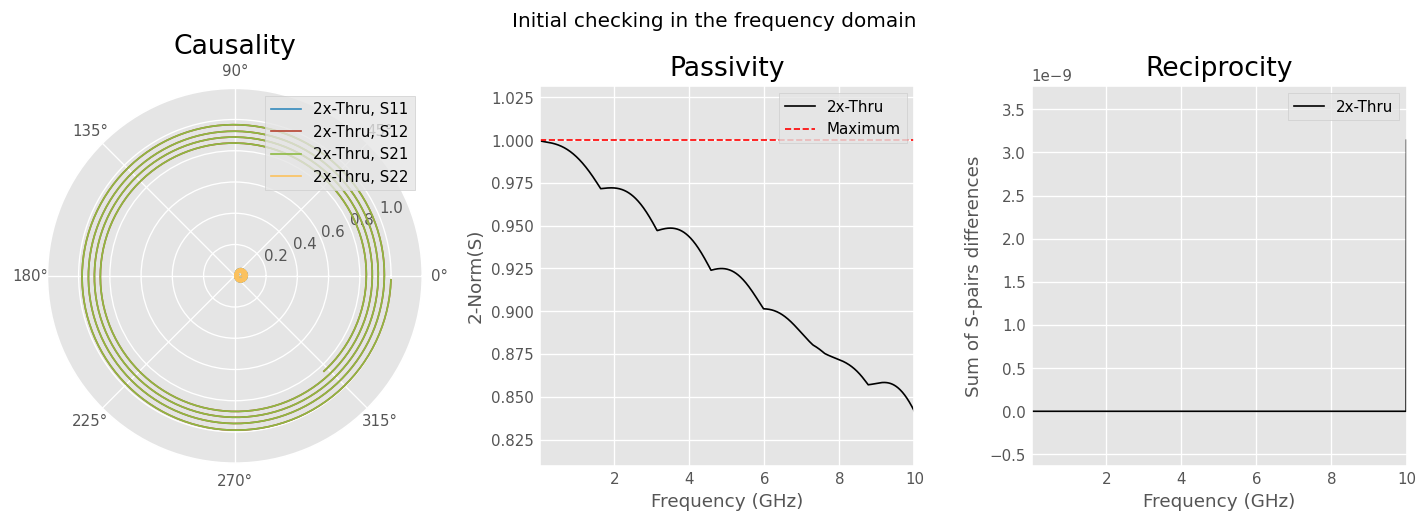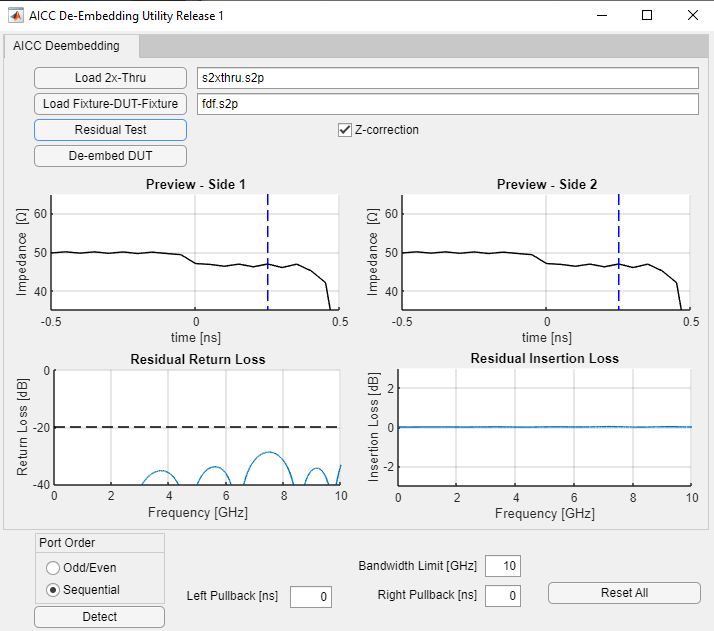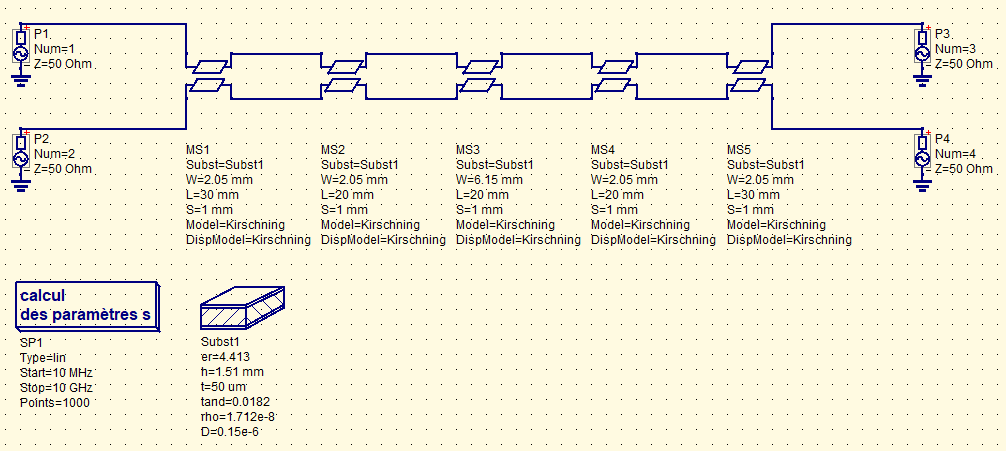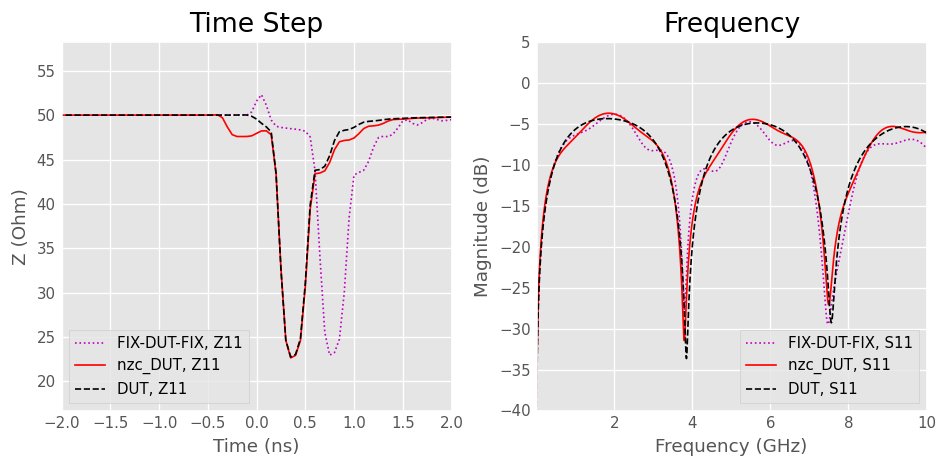# IEEEP370 Deembedding¶

## Introduction¶

The IEEEP370 standards provides good practices for ensuring the quality of measured data for high-frequency electrical interconnects at frequencies up to 50 GHz. It recommends methods and processes for ensuring the accuracy and consistency of measured data for signals with frequency content up to 50 GHz, in particular for removing test fixture and instrumentation effects.

In the examples below, and following the standard proposal on labelling, a 2-port DUT have one fixture attached to each of its port. The fixture attached to the single-ended DUT port 1 is labeled as FIX-1. The fixture attached to the single-ended DUT port 2 is labeled as FIX-2. The name of the fixture is shortened to FIX and the composite structure as FIX-DUT-FIX. The combination of the DUT and fixtures on either end is referred to as the composite structure or the FIX-DUT-FIX structure.The combination of just the two fixtures, such as a FIX-1 connected to a FIX-2, is referred to as a FIX-FIX structure or a 2x-Thru.The purpose of this notebook is to illustrate the scikit-rf implementation of the deembedding methods proposed within the IEEE P370 standard to remove the test fixture effects.

First, let’s make the necessary Python import statements:

:

import skrf as rf
import matplotlib.pyplot as plt
import numpy as np
from skrf.calibration import (IEEEP370_SE_NZC_2xThru, IEEEP370_MM_NZC_2xThru,
IEEEP370_SE_ZC_2xThru, IEEEP370_MM_ZC_2xThru)
from skrf.media import MLine
rf.stylely()


## Single-ended¶

The algorithms can be used with 2-port single-ended networks.

### Simulation of 2xThru, DUT and Fixture-DUT-Fixture¶

We use scikit-rf MLine media to simulate microstrip lines artifacts. This is convenient to vary parameters without leaving Python to operate another software to generate s-parameter files.

• dut is a Beatty structure with a 3xWidth microstrip segment connected left and right by two uniform 1xWidth microstrip.

• fdf is FIX-DUT-FIX, prolongating the DUT left and right lines (connector and launch not simulated).

• s2xthru is FIX-FIX and is the two prolongating lines connected in the middle without the DUT. For example purpose the width is changed by 20% to show the effect of such a mismatch in the deembedding process.

The target is to bisect s2xthru to get the left and right fixtures models and get DUT by deembedding from FIX-DUT-FIX.

The microstrip lines are inspired by this example.

:

directory = 'ieeep370deembedding/'
freq = rf.F(1e-3,10,10000, 'ghz')
W   = 3.20e-3 #3.00e-3 for 50 ohm
H   = 1.55e-3
T   = 50e-6
ep_r = 4.459
tanD = 0.0183
f_epr_tand = 1e9

# microstrip segments
MSL1 = MLine(frequency=freq, z0=50, w=W, h=H, t=T,
ep_r=ep_r, mu_r=1, rho=1.712e-8, tand=tanD, rough=0.15e-6,
f_low=1e3, f_high=1e12, f_epr_tand=f_epr_tand,
diel='djordjevicsvensson', disp='kirschningjansen')

# capacitive 3 x width Beatty structure
MSL2 = MLine(frequency=freq, z0=50, w=3*W, h=H, t=T,
ep_r=ep_r, mu_r=1, rho=1.712e-8, tand=tanD, rough=0.15e-6,
f_low=1e3, f_high=1e12, f_epr_tand=f_epr_tand,
diel='djordjevicsvensson', disp='kirschningjansen')

# microstrip segment with a 20% variation of width
MSL3 = MLine(frequency=freq, z0=50, w=0.8*W, h=H, t=T,
ep_r=ep_r, mu_r=1, rho=1.712e-8, tand=tanD, rough=0.15e-6,
f_low=1e3, f_high=1e12, f_epr_tand=f_epr_tand,
diel='djordjevicsvensson', disp='kirschningjansen')

# building DUT
dut =    MSL1.line(20e-3, 'm', embed=True, z0=MSL1.Z0) \
** MSL2.line(20e-3, 'm', embed=True, z0=MSL2.Z0) \
** MSL1.line(20e-3, 'm', embed=True, z0=MSL1.Z0)
dut.name = 'DUT'

# building FIXTURE-DUT-FIXTURE
thru1 = MSL1.line(20e-3, 'm', embed=True, z0=MSL1.Z0)
thru3 = MSL3.line(20e-3, 'm', embed=True, z0=MSL3.Z0)
fdf     = thru1 ** dut ** thru1
fdf.name = 'FIX-DUT-FIX'

# building FIXTURE-FIXTURE with a 20% width variation from FIXTURE-DUT-FIXTURE(2xthru)
s2xthru = thru3 ** thru3
s2xthru.name = '2x-Thru'

# extrapolate to DC for time step
dut_dc = dut.extrapolate_to_dc(kind='cubic')
fdf_dc = fdf.extrapolate_to_dc(kind='cubic')
s2xthru_dc = s2xthru.extrapolate_to_dc(kind='cubic')
# set True to write .s2p files
if False:
s2xthru.write_touchstone(directory + 'se_2xthru.s2p')
fdf.write_touchstone(directory + 'se_fdf.s2p')

/home/docs/checkouts/readthedocs.org/user_builds/scikit-rf/envs/latest/lib/python3.8/site-packages/skrf/media/mline.py:631: RuntimeWarning: Conductor loss calculation invalid for lineheight t (5e-05)  < 3 * skin depth (6.585246128681408e-05)
warnings.warn(
/tmp/ipykernel_2030/809007891.py:29: FutureWarning: In a future version,embed will be deprecated.
The line and media port impedance z0 and characteristic impedance Z0 will be used instead to determine if the line has to be renormalized.
dut =    MSL1.line(20e-3, 'm', embed=True, z0=MSL1.Z0) \
/tmp/ipykernel_2030/809007891.py:30: FutureWarning: In a future version,embed will be deprecated.
The line and media port impedance z0 and characteristic impedance Z0 will be used instead to determine if the line has to be renormalized.
** MSL2.line(20e-3, 'm', embed=True, z0=MSL2.Z0) \
/tmp/ipykernel_2030/809007891.py:31: FutureWarning: In a future version,embed will be deprecated.
The line and media port impedance z0 and characteristic impedance Z0 will be used instead to determine if the line has to be renormalized.
** MSL1.line(20e-3, 'm', embed=True, z0=MSL1.Z0)
/tmp/ipykernel_2030/809007891.py:35: FutureWarning: In a future version,embed will be deprecated.
The line and media port impedance z0 and characteristic impedance Z0 will be used instead to determine if the line has to be renormalized.
thru1 = MSL1.line(20e-3, 'm', embed=True, z0=MSL1.Z0)
/tmp/ipykernel_2030/809007891.py:36: FutureWarning: In a future version,embed will be deprecated.
The line and media port impedance z0 and characteristic impedance Z0 will be used instead to determine if the line has to be renormalized.
thru3 = MSL3.line(20e-3, 'm', embed=True, z0=MSL3.Z0)


Looking at generated networks. The _dc networks were extraploated to DC to enable time step plot. The time shift of dut caused be the fixture and the 2xthru impedance mismatch are clearly visibles.

:

plt.figure(figsize=(10, 5))
plt.subplot(1, 2, 1)
plt.title('Time Step')
dut_dc.s11.plot_z_time_step(window='hamming')
fdf_dc.s11.plot_z_time_step(window='hamming')
s2xthru_dc.s11.plot_z_time_step(window='hamming')
plt.xlim((-2, 2))
plt.ylim((15, 55))
plt.legend(loc = 'lower left')
plt.subplot(1, 2, 2)
plt.title('Frequency')
dut.s11.plot_s_db()
fdf.s11.plot_s_db()
s2xthru.s11.plot_s_db()### IEEEP370_SE_NZC_2xthru without impedance correction¶

This method only takes 2x-Thru as input. It is quite simple and efficient but cannot correct for the difference of impedance between the lines of FIX-FIX and FIX-DUT-FIX. Of course this difference is not wanted, but it could occurs depending manufacturing process or if the artefacts are not built on the same board.

IEEEP370 recommend following consistency checks:

• Self de-embedding of 2x-Thru with absolute magnitude of residual insertion loss < 0.1 dB and phase < 1 degree

• Compare the TDR of the fixture model to the FIX-DUT-FIX

:

dm_nzc = IEEEP370_SE_NZC_2xThru(dummy_2xthru = s2xthru, name = '2xthru')
nzc_fix1 = dm_nzc.s_side1
nzc_fix1.name = 'nzc_FIX-1'
nzc_fix2 = dm_nzc.s_side2
nzc_fix2.name = 'nzc_FIX-2'
nzc_d_dut = dm_nzc.deembed(fdf)
nzc_d_dut.name = 'nzc_DUT'
nzc_fix1_dc = nzc_fix1.extrapolate_to_dc(kind='cubic')
nzc_d_dut_dc = nzc_d_dut.extrapolate_to_dc(kind='cubic')

# compute residuals
res_nzc = dm_nzc.deembed(s2xthru)
res_nzc.name = 'nzc_residuals'
res_nzc.s += 1e-15 # avoid numeric singularities

# plot them all
plt.figure(figsize=(10, 10))
plt.subplot(2, 2, 1)
plt.title('Time Step')
dut_dc.s11.plot_z_time_step(window='hamming')
nzc_d_dut_dc.s11.plot_z_time_step(window='hamming')
fdf_dc.s11.plot_z_time_step(window='hamming')
s2xthru_dc.s11.plot_z_time_step(window='hamming')
nzc_fix1_dc.s11.plot_z_time_step(window='hamming')
plt.xlim((-2, 2))
plt.ylim((15, 55))
plt.legend(loc = 'lower left')
plt.subplot(2, 2, 2)
plt.title('Frequency')
dut.plot_s_db(0, 0)
nzc_d_dut.plot_s_db(0, 0)
fdf.plot_s_db(0, 0)
plt.ylim((-40, 5))
plt.subplot(2, 2, 3)
res_nzc.plot_s_db(1,0)
plt.subplot(2, 2, 4)
res_nzc.plot_s_deg(1,0)The NZC deembedding has removed the delay of the fixture but, as expected, the difference of impedance between FIX-FIX and FIX-DUT-FIX are causing an impedance bounce in timestep.

However, the agreement between fixtures models and 2xthru is excellent, as shown by magnitude and phase of residuals being much smaller than IEEEP370 ±0.1 dB and ±1° limits.

### IEEEP370_SE_ZC_2xThru with impedance correction¶

This method take 2x-Thru and FIX-DUT-FIX as inputs. It makes a correction for the (unwanted) difference of impedance between the lines of FIX-FIX and FIX-DUT-FIX.

:

dm_zc  = IEEEP370_SE_ZC_2xThru(dummy_2xthru = s2xthru, dummy_fix_dut_fix = fdf,
bandwidth_limit = 10e9, pullback1 = 0, pullback2 = 0,
leadin = 0, NRP_enable = False,
name = 'zc2xthru')
zc_d_dut = dm_zc.deembed(fdf)
zc_d_dut.name = 'zc_DUT'
zc_fix1 = dm_zc.s_side1
zc_fix1.name = 'zc_FIX-1'
zc_fix2 = dm_zc.s_side2
zc_fix2.name = 'zc_FIX-2'
zc_side1_dc = zc_fix1.extrapolate_to_dc(kind='cubic')
zc_d_dut_dc = zc_d_dut.extrapolate_to_dc(kind='cubic')

# compute residuals
res_zc = dm_zc.deembed(s2xthru)
res_zc.name = 'zc_residuals'
res_zc.s += 1e-15 # avoid numeric singularities

# plot them all
plt.figure(figsize=(10, 10))
plt.subplot(2, 2, 1)
plt.title('Time Step')
dut_dc.s11.plot_z_time_step(window='hamming')
zc_d_dut_dc.s11.plot_z_time_step(window='hamming')
fdf_dc.s11.plot_z_time_step(window='hamming')
s2xthru_dc.s11.plot_z_time_step(window='hamming')
zc_side1_dc.s11.plot_z_time_step(window='hamming')
plt.xlim((-2, 2))
plt.ylim((15, 55))
plt.legend(loc = 'lower left')
plt.subplot(2, 2, 2)
plt.title('Frequency')
dut.plot_s_db(0, 0)
zc_d_dut.plot_s_db(0, 0)
fdf.plot_s_db(0, 0)
plt.ylim((-40, 5))
plt.subplot(2, 2, 3)
res_zc.plot_s_db(1,0)
plt.subplot(2, 2, 4)
res_zc.plot_s_deg(1,0)As expected, the ZC deembedding shows a better agreement on time step than NZC. This is because ZC compensates the slight impedance difference between FIX-FIX and FIX-DUT-FIX of this example. Of course, this difference should be minimized as much as possible at the manufacturing stage.

While the agreement of time domain is better thanks to impedance correction, the mismatch causes the residuals magnitude of fixture and 2xthru to be more significant but almost fit into IEEEP370 ±0.1 dB limit. This is because of the impedance mismatch between fixture and 2x-Thru. The residual phase is kept within the IEEEP370 limits of ±1°, which is not bad.

### Single Ended Comparison with AICC De-Embedding Utility¶

A set of reference Matlab or Octave codes that implement the IEEEP370 NZC and ZC deembedding algorithms are available with an open source BSD-3-Clause license on IEEE repo

However, not everyone has access to Matlab and RF Toolbox. Maybe, is this one of the reasons why you are reading this text and looking forward to using scikit-rf and Python.

A compiled binary of Matlab routine with a gui is available on Amphenol website with the name “ACS De-embedding Utility”.Let’s compare the output of this tool on scikit-rf port of deembedding algorithms as a consistency-check.

:

# read AICC generated files
nzc_ref = rf.Network(directory + 'deembedded_SE_NZC_se_fdf.s2p')
zc_ref = rf.Network(directory + 'deembedded_SE_ZC_se_fdf.s2p')
nzc_ref_dc = nzc_ref.extrapolate_to_dc(kind='cubic')
zc_ref_dc = zc_ref.extrapolate_to_dc(kind='cubic')

# compute residuals
res_nzc2 = nzc_d_dut.inv ** nzc_ref
res_zc2 = zc_d_dut.inv ** zc_ref

# plot them all
plt.figure(figsize=(10, 10))
plt.subplot(2, 2, 1)
plt.title('NZC Time Step')
nzc_d_dut_dc.s11.plot_z_time_step(window='hamming', marker = 'd', linestyle='None')
nzc_ref_dc.s11.plot_z_time_step(window='hamming')
plt.legend(loc = 'lower left')
plt.xlim((-2, 2))
plt.ylim((15, 55))
plt.subplot(2, 2, 2)
plt.title('ZC Time Step')
zc_d_dut_dc.s11.plot_z_time_step(window='hamming', marker = 's', linestyle='None')
zc_ref_dc.s11.plot_z_time_step(window='hamming')
plt.legend(loc = 'lower left')
plt.xlim((-2, 2))
plt.ylim((15, 55))
plt.legend(loc = 'lower left')
plt.subplot(2, 2, 3)
res_nzc2.plot_s_db(1,0)
res_zc2.plot_s_db(1,0)
plt.subplot(2, 2, 4)
res_nzc2.plot_s_deg(1,0)
res_zc2.plot_s_deg(1,0)Both NZC and ZC show visually a good agreement in time domain plots. The residuals phase and magnitude of NZC against AICC tool are small according to IEEEP370 limits. The magnitude and phase of ZC dare within the 0.1 dB and 1° limit up to 9 GHz. The small high-frequency deviation would not affect the time step shape very much.

In conclusion, for the specific single-ended case studied, we can say that scikit-rf give consistent results with AICC De-embedding Utility implementation if IEEEP370 deembedding routines.

## Mixed mode¶

ZC and NZC deembedding can also be used on differential 4-port measurements thanks to single-ended to generalized mixed mode parameters transformation. Two Thrus are modeled, respectively for differential and for common modes and mixed back into a single-ended fixture model that can be deembedded from measurement.

### Simulation of 2xThru, DUT and Fixture-DUT-Fixture¶

We use Qucs to simulate coupled microstrip lines artifacts. This is a free simulator that can generate s-parameters of equation-based RF devices, among other things.

• diff_dut is a Beatty structure with a 3xWidth segment connected left and right by two uniform 1xWidth coupled microstrip.

• diff_fdf is Fixture-DUT-Fixture, prolongating the DUT left and right lines (connector and launch not simulated).

• diff_2xthru is Fixture-Fixture and is the two prolongating lines connected in the middle without the DUT. For example purpose the width is changed by 20% to show the effect of such a mismatch in the deembedding process.The files as well as qucs sources are located in the directory ieeep370deembedding next to this notebook.

### IEEEP370_MM_NZC_2xThru without impedance correction¶

This method only takes 2x-Thru as input. It is quite simple and efficient but cannot correct for the difference of impedance between the lines of FIX-FIX and FIX-DUT-FIX. Of course this difference is not wanted, but it could occurs depending manufacturing process or if the artefacts are not built on the same board.

:

# load single-ended data
se_ref_nzc = rf.Network(directory + 'deembedded_MM_NZC_diff_fdf.s4p')
se_dut = rf.Network(directory + 'diff_dut.s4p')
se_2xthru = rf.Network(directory + 'diff_2xthru.s4p')
se_fdf = rf.Network(directory + 'diff_fdf.s4p')

# transform to mixed-modes
mm_ref_nzc = se_ref_nzc.copy()
mm_ref_nzc.se2gmm(p = 2)
mm_2xthru = se_2xthru.copy()
mm_2xthru.se2gmm(p = 2)
mm_fdf = se_fdf.copy()
mm_fdf.se2gmm(p = 2)
mm_dut = se_dut.copy()
mm_dut.se2gmm(p = 2)

# extrapolate to DC for time step
mm_2xthru_dc = mm_2xthru.extrapolate_to_dc(kind='cubic')
mm_ref_nzc_dc = mm_ref_nzc.extrapolate_to_dc(kind='cubic')
mm_dut_dc = mm_dut.extrapolate_to_dc(kind='cubic')
mm_fdf_dc = mm_fdf.extrapolate_to_dc(kind='cubic')

# de-embedding
z0 = 50
dm_mmnzc  = IEEEP370_MM_NZC_2xThru(dummy_2xthru = se_2xthru, z0 = z0, name = 'mmnzc')
se_d_dut_nzc = dm_mmnzc.deembed(se_fdf)
mm_d_dut_nzc = se_d_dut_nzc.copy()
mm_d_dut_nzc.se2gmm(p = 2)
mm_nzc_side1 = dm_mmnzc.se_side1.copy()
mm_nzc_side1.se2gmm(p = 2)
mm_d_dut_nzc_dc = mm_d_dut_nzc.extrapolate_to_dc(kind='cubic')
mm_nzc_side1_dc = mm_nzc_side1.extrapolate_to_dc(kind='cubic')

# residuals for consistency check
mm_res_nzc = dm_mmnzc.deembed(se_2xthru)
mm_res_nzc.se2gmm(p = 2)
mm_res_nzc.s += 1e-15 # avoid numeric singularities
mm_res_nzc_dc = mm_res_nzc.extrapolate_to_dc(kind='cubic')

# plot them all
plt.figure(figsize=(10, 10))
plt.subplot(2, 1, 1)
plt.title('MM NZC Time Step')
mm_dut_dc.s11.plot_z_time_step(window='hamming', z0 = np.real(mm_dut_dc.z0[0, 0]), color = 'k', marker = 's', linestyle='None', label = 'DUT, sdd11')
mm_dut_dc.s33.plot_z_time_step(window='hamming', z0 = np.real(mm_dut_dc.z0[2, 2]), color = 'k', marker = 's', linestyle='None', label = 'DUT, scc11')
mm_d_dut_nzc_dc.s11.plot_z_time_step(window='hamming', z0 = np.real(mm_d_dut_nzc_dc.z0[0, 0]), color = 'r', label = 'nzc_DUT, sdd11')
mm_d_dut_nzc_dc.s33.plot_z_time_step(window='hamming', z0 = np.real(mm_d_dut_nzc_dc.z0[2, 2]), color = 'r', label = 'nzc_DUT, scc11')
mm_fdf_dc.s11.plot_z_time_step(window='hamming', z0 = np.real(mm_fdf_dc.z0[0, 0]), color = 'g', label = 'FIX-DUT-FIX, sdd11')
mm_fdf_dc.s33.plot_z_time_step(window='hamming', z0 = np.real(mm_fdf_dc.z0[2, 2]), color = 'g', label = 'FIX-DUT-FIX, scc11')
mm_2xthru_dc.s11.plot_z_time_step(window='hamming', z0 = np.real(mm_2xthru_dc.z0[0, 0]), color = 'c', label = '2x-Thru, sdd11')
mm_2xthru_dc.s33.plot_z_time_step(window='hamming', z0 = np.real(mm_2xthru_dc.z0[2, 2]), color = 'c', label = '2x-Thru, scc11')
mm_nzc_side1_dc.s11.plot_z_time_step(window='hamming', z0 = np.real(mm_nzc_side1_dc.z0[0, 0]), color = 'b', label = 'FIX-1_2, sdd11')
mm_nzc_side1_dc.s33.plot_z_time_step(window='hamming', z0 = np.real(mm_nzc_side1_dc.z0[2, 2]), color = 'b', label = 'FIX-1_2, scc11')
plt.xlim((-1, 2))
plt.legend(loc='lower right')
plt.subplot(2, 2, 3)
mm_res_nzc.plot_s_db(1, 0, label = 'residuals, sdd11')
mm_res_nzc.plot_s_db(3, 2, label = 'residuals, scc11')
plt.subplot(2, 2, 4)
mm_res_nzc.plot_s_deg(1, 0, label = 'residuals, sdd11')
mm_res_nzc.plot_s_deg(3, 2, label = 'residuals, scc11')As expected, the impedance mismatch between 2xthru and FIX-DUT-FIX cause an impedance bounce in deembedded DUT.

Residuals computed by deembedding the 2xthru to check consistency shows results that are very small both in magnitude and phase. Both are within the IEEEP370 ±0.1 dB and ±1° limits on full bandwidth.

### IEEEP370_MM_ZC_2xThru with impedance correction¶

This method take 2x-Thru and FIX-DUT-FIX as inputs. It makes a correction for the (unwanted) difference of impedance between the lines of FIX-FIX and FIX-DUT-FIX.

:

# load single-ended data
se_ref_zc = rf.Network(directory + 'deembedded_MM_ZC_diff_fdf.s4p')

# transform to mixed-modes
mm_ref_zc = se_ref_zc.copy()
mm_ref_zc.se2gmm(p = 2)

# extrapolate to DC for time step
mm_ref_zc_dc = mm_ref_zc.extrapolate_to_dc(kind='cubic')

# de-embedding
dm_mmzc  = IEEEP370_MM_ZC_2xThru(dummy_2xthru = se_2xthru, dummy_fix_dut_fix = se_fdf,
bandwidth_limit = 10e9, pullback1 = 0, pullback2 = 0,
leadin = 0, NRP_enable = False, name = 'mmzc')
se_d_dut_zc = dm_mmzc.deembed(se_fdf)
mm_d_dut_zc = se_d_dut_zc.copy()
mm_d_dut_zc.se2gmm(p = 2)
mm_zc_side1 = dm_mmzc.se_side1.copy()
mm_zc_side1.se2gmm(p = 2)
mm_d_dut_zc_dc = mm_d_dut_zc.extrapolate_to_dc(kind='cubic')
mm_zc_side1_dc = mm_zc_side1.extrapolate_to_dc(kind='cubic')

# residuals for consistency check
mm_res_zc = dm_mmzc.deembed(se_2xthru)
mm_res_zc.se2gmm(p = 2)
mm_res_zc.s += 1e-15 # avoid numeric singularities
mm_res_zc_dc = mm_res_zc.extrapolate_to_dc(kind='cubic')

# plot them all
plt.figure(figsize=(10, 10))
plt.subplot(2, 1, 1)
plt.title('MM ZC Time Step')
mm_dut_dc.s11.plot_z_time_step(window='hamming', z0 = np.real(mm_dut_dc.z0[0, 0]), color = 'k', marker = 's', linestyle='None', label = 'DUT, sdd11')
mm_dut_dc.s33.plot_z_time_step(window='hamming', z0 = np.real(mm_dut_dc.z0[2, 2]), color = 'k', marker = 's', linestyle='None', label = 'DUT, scc11')
mm_d_dut_zc_dc.s11.plot_z_time_step(window='hamming', z0 = np.real(mm_d_dut_zc_dc.z0[0, 0]), color = 'r', label = 'zc_DUT, sdd11')
mm_d_dut_zc_dc.s33.plot_z_time_step(window='hamming', z0 = np.real(mm_d_dut_zc_dc.z0[2, 2]), color = 'r', label = 'zc_DUT, scc11')
mm_fdf_dc.s11.plot_z_time_step(window='hamming', z0 = np.real(mm_fdf_dc.z0[0, 0]), color = 'g', label = 'FIX-DUT-FIX, sdd11')
mm_fdf_dc.s33.plot_z_time_step(window='hamming', z0 = np.real(mm_fdf_dc.z0[2, 2]), color = 'g', label = 'FIX-DUT-FIX, scc11')
mm_2xthru_dc.s11.plot_z_time_step(window='hamming', z0 = np.real(mm_2xthru_dc.z0[0, 0]), color = 'c', label = '2x-Thru, sdd11')
mm_2xthru_dc.s33.plot_z_time_step(window='hamming', z0 = np.real(mm_2xthru_dc.z0[2, 2]), color = 'c', label = '2x-Thru, scc11')
mm_zc_side1_dc.s11.plot_z_time_step(window='hamming', z0 = np.real(mm_zc_side1_dc.z0[0, 0]), color = 'b', label = 'FIX-1_2, sdd11')
mm_zc_side1_dc.s33.plot_z_time_step(window='hamming', z0 = np.real(mm_zc_side1_dc.z0[2, 2]), color = 'b', label = 'FIX-1_2, scc11')
plt.xlim((-1, 2))
plt.legend(loc='lower right')
plt.subplot(2, 2, 3)
mm_res_zc.plot_s_db(1, 0, label = 'residuals, sdd11')
mm_res_zc.plot_s_db(3, 2, label = 'residuals, scc11')
plt.subplot(2, 2, 4)
mm_res_zc.plot_s_deg(1, 0, label = 'residuals, sdd11')
mm_res_zc.plot_s_deg(3, 2, label = 'residuals, scc11')The plot show that the impedance mismatch between 2x-Thru and FIX-DUT-FIX has been corrected for the computation of fixture side1.

The phase and magnitude residuals are out from the ±0.1 dB and ±1° boundaries, but not from that much.

### Mixed Mode Comparison with AICC De-Embedding Utility¶

Let’s compare the deembedded DUTs from scikit-rf and AICC De-Embedding Utility to conclude this notebook.

:

mm_nzc_residuals = se_d_dut_nzc.inv ** se_ref_nzc
mm_nzc_residuals.se2gmm(p = 2)
mm_nzc_residuals.s += 1e-15 # avoid numeric singularities
mm_zc_residuals = se_d_dut_zc.inv ** se_ref_zc
mm_zc_residuals.se2gmm(p = 2)
mm_zc_residuals.s += 1e-15 # avoid numeric singularities

# plot them all
plt.figure(figsize = (10, 10))
plt.subplot(2, 2, 1)
plt.title('AICC Tool Time Comparison')
mm_ref_nzc_dc.s11.plot_z_time_step(window='hamming', z0 = np.real(mm_ref_nzc_dc.z0[0, 0]), label = 'aicc_nzc, sdd11')
mm_ref_nzc_dc.s33.plot_z_time_step(window='hamming', z0 = np.real(mm_ref_nzc_dc.z0[2, 2]), label = 'aicc_nzc, scc11')
mm_d_dut_nzc_dc.s11.plot_z_time_step(window='hamming', z0 = np.real(mm_d_dut_nzc_dc.z0[0, 0]), marker = 's', linestyle='None', label = 'd_dut_nzc, sdd11')
mm_d_dut_nzc_dc.s33.plot_z_time_step(window='hamming', z0 = np.real(mm_d_dut_nzc_dc.z0[2, 2]), marker = 's', linestyle='None', label = 'd_dut_nzc, scc11')
plt.xlim((-1, 2))
plt.subplot(2, 2, 2)
plt.title('AICC Tool Time Comparison')
mm_ref_zc_dc.s11.plot_z_time_step(window='hamming', z0 = np.real(mm_ref_zc_dc.z0[0, 0]), label = 'aicc_zc, sdd11')
mm_ref_zc_dc.s33.plot_z_time_step(window='hamming', z0 = np.real(mm_ref_zc_dc.z0[2, 2]), label = 'aicc_zc, scc11')
mm_d_dut_zc_dc.s11.plot_z_time_step(window='hamming', z0 = np.real(mm_d_dut_zc_dc.z0[0, 0]), marker = 's', linestyle='None', label = 'd_dut_zc, sdd11')
mm_d_dut_zc_dc.s33.plot_z_time_step(window='hamming', z0 = np.real(mm_d_dut_zc_dc.z0[2, 2]), marker = 's', linestyle='None', label = 'd_dut_zc, scc11')
plt.xlim((-1, 2))
plt.subplot(2, 2, 3)
plt.title('Magnitude Residuals')
mm_nzc_residuals.plot_s_db(1, 0, label = 'NZC, sdd11')
mm_nzc_residuals.plot_s_db(3, 2, label = 'NZC, scc11')
mm_zc_residuals.plot_s_db(1, 0, label = 'ZC, sdd11')
mm_zc_residuals.plot_s_db(3, 2, label = 'ZC, scc11')
plt.subplot(2, 2, 4)
plt.title('Phase Residuals')
mm_nzc_residuals.plot_s_deg(1, 0, label = 'NZC, sdd11')
mm_nzc_residuals.plot_s_deg(3, 2, label = 'NZC, scc11')
mm_zc_residuals.plot_s_deg(1, 0, label = 'ZC, sdd11')
mm_zc_residuals.plot_s_deg(3, 2, label = 'ZC, scc11')The agreement between scikit-rf and AICC De-Embedding Utility is good both visually in time domain and on magnitude and phase residuals that are within ±0.4 dB and ±2° on full bandwidth. The agreement is even better for the NZC algorithm.

In conclusion, for the specific mixed mode case studied, we can say that scikit-rf gives reasonably consistent results with AICC De-embedding Utility implementation in IEEEP370 deembedding routines.

[ ]: